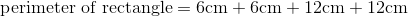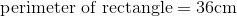# ISEE Middle Level Math : How to find the perimeter of the rectangle

## Example Questions

### Example Question #11 : How To Find The Perimeter Of The Rectangle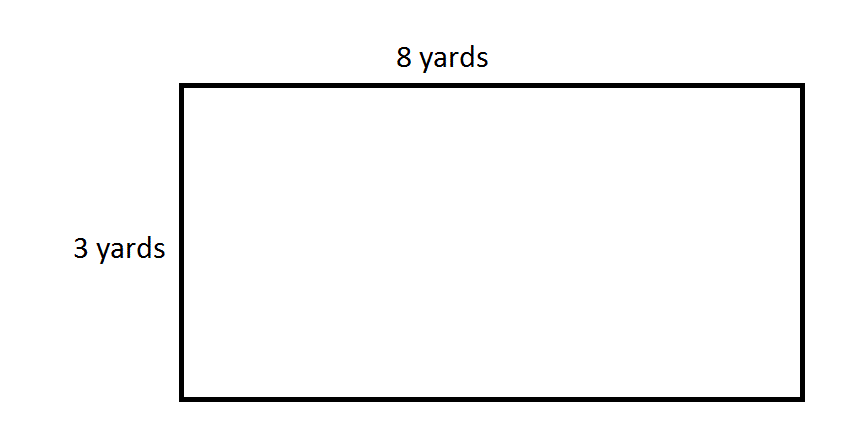Give the perimeter of the above rectangle in centimeters, using the conversion factor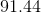centimeters per yard.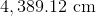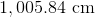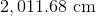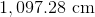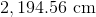Explanation:

The perimeter of the rectangle is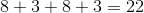yards. To convert this to centimeters, multiply by the given conversion factor: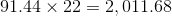centimeters.

### Example Question #12 : How To Find The Perimeter Of The Rectangle

Find the perimeter of a rectangle whose length is 6 and width is 5.Explanation:

To solve, simply use the formula for the perimeter of a rectangle.

In this particular case the length and width are,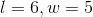Thus,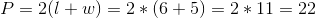### Example Question #13 : How To Find The Perimeter Of The Rectangle

rectangle has a length of 9 centimeters and a width of 5 centimeters. Find the perimeter.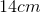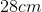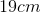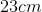Explanation:

The formula for the perimeter of a rectangle is: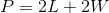where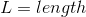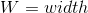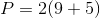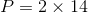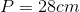### Example Question #14 : How To Find The Perimeter Of The Rectangle

The length of a rectangle is 1 cm more than 3 times the width.  What is the perimeter of the rectangle if the width is 32 cm?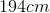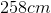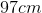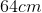Explanation:

First find the length.

length or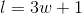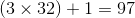The length isFind the perimeter.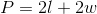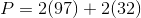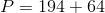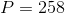The perimeter iswhen the width is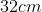.

### Example Question #15 : How To Find The Perimeter Of The Rectangle

Use the following to answer the question.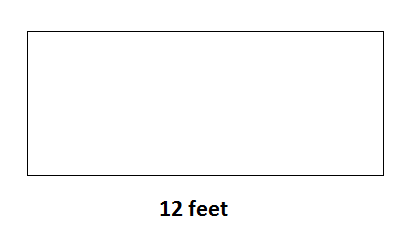Find the perimeter of the rectangle if the width is half the length.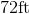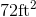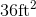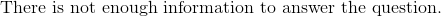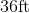Explanation:

To find the perimeter of a rectangle, we use the following formula: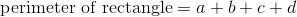where a, b, c, and d are the lengths of the sides of the rectangle.

Now, in the rectanglewe can see the length is 12 feet.  Because it is a rectangle, we know the opposite side is also 12 feet.  We know the width is half of the length.  So, the width is 6 feet.  Because it is a rectangle, the opposite side is also 6 feet.  Knowing this, we can substitute into the formula.  We get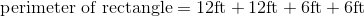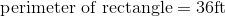### Example Question #16 : How To Find The Perimeter Of The Rectangle

The Smartboard in your math classroom is 3 feet tall and 20 feet long. What is the perimeter of the Smartboard?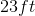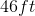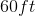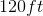Explanation:

The Smartboard in your math classroom is 3 feet tall and 20 feet long. What is the perimeter of the Smartboard?

We need to find the perimeter of the rectangle that is the Smartboard.

To do so, we simply add up the sides. In this case, we have 2 3ft sides and 2 20 ft sides.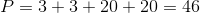### Example Question #17 : How To Find The Perimeter Of The Rectangle

Find the distance around a rug if the rug is 2 feet wide and its length is 3 times the width.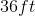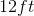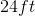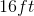Explanation:

Find the distance around a rectangular rug if the rug is 2 feet wide and its length is 3 times the width.

We are really being asked to find the perimeter. To do so, we first need to dimensions.

We are told the width is two feet.

The length is three times the width.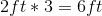Now, use the following to find the perimeter: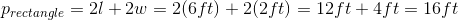So our answer is 16 feet

### Example Question #18 : How To Find The Perimeter Of The Rectangle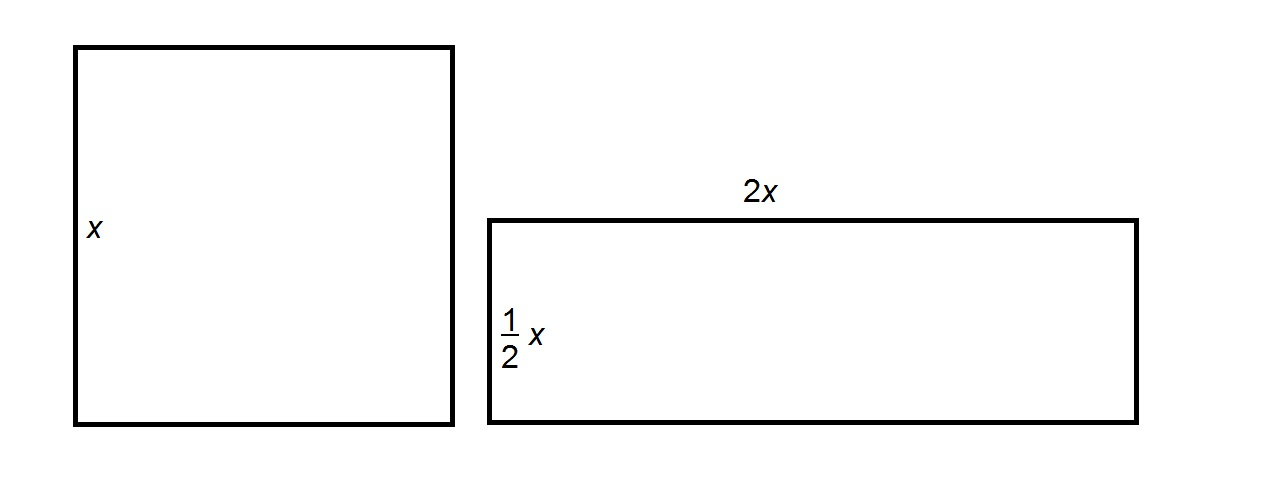Refer to the above figures. The square at left has perimeter 160. Give the perimeter of the rectangle at right.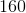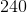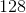Explanation:

The perimeter of a square is four times the length of a side - here, this is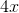.

The perimeter of a rectangle is twice the sum of its length and its width - here, this is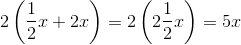.

The square has perimeter 160, so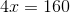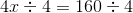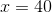The perimeter of the rectangle is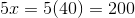.

### Example Question #19 : How To Find The Perimeter Of The Rectangle

A rectangular postage stamp has a width of 3 cm and a height of 12 cm. Find the perimeter of the stamp.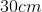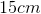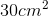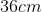Explanation:

A rectangular postage stamp has a width of 3 cm and a height of 12 cm. Find the perimeter of the stamp.

To find the perimeter of a rectangle (or any shape) simply add up all the sides.

In this case, we have two sides that are 3 cm and two sides that are 12 cm.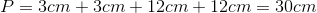Now, our answer must be in centimeters and not centimeters squared, because we are dealing with perimeter, which is a measure of length.

### Example Question #20 : How To Find The Perimeter Of The Rectangle

Find the perimeter of a rectangle with a width of 6 cm and a length that is two times the width.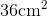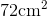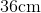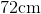Explanation:

To find the perimeter of a rectangle, we will use the following formula:where a, b, c, and d are the lengths of the sides of the rectangle.

Now, we know the width of the rectangle is 6cm.  Because it is a rectangle, we know the opposite side is equal.  Therefore, the opposite side is also 6cm.

We also know the length is two times the width.  Therefore, the length is 12cm.  Because it is a rectangle, we know the opposite side is equal.  Therefore, the opposite side is also 12cm.

Knowing this, we can substitute into the formula.  We get